# Sample Data: Fin Pines

Locations of pine trees annotated with diameter (in centimeters) and height (in meters) marks

## Details

Locations of pine trees in the observation region Rectangle[{-5,-8}, {5,2}] meters, annotated with diameter (in centimeters) and height (in meters) marks.

## Examples

### Basic Examples (1)

 In:=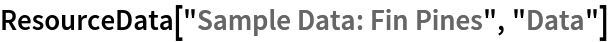Out=Summary of the spatial point data:

 In:=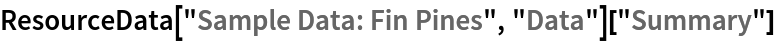Out=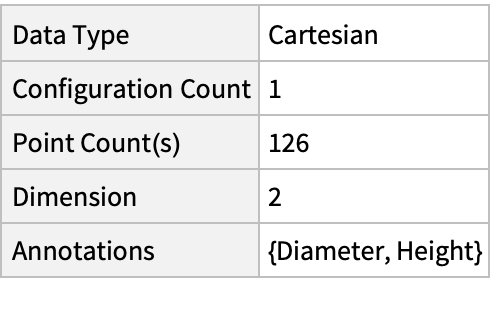### Visualizations (1)

 In:=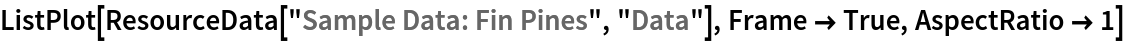Out=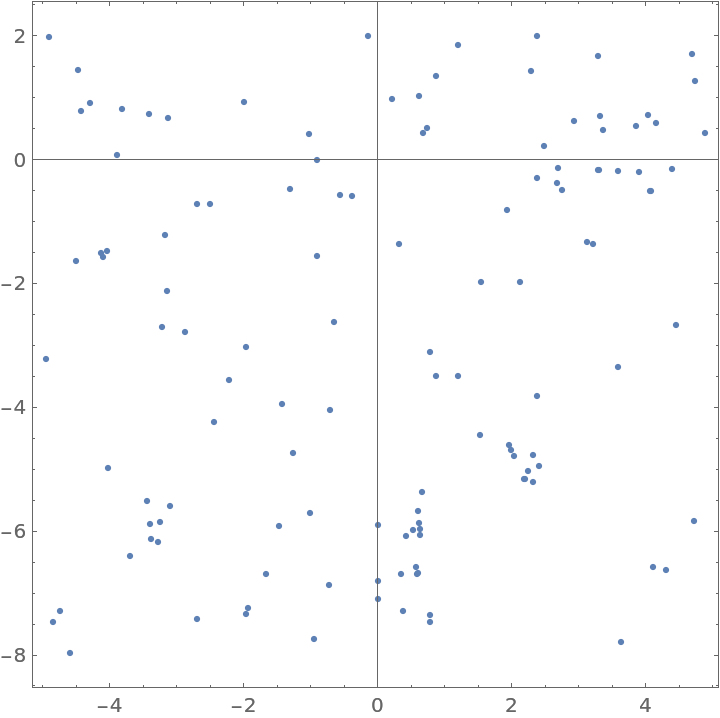Visualize points with diameter annotations:

 In:=Out=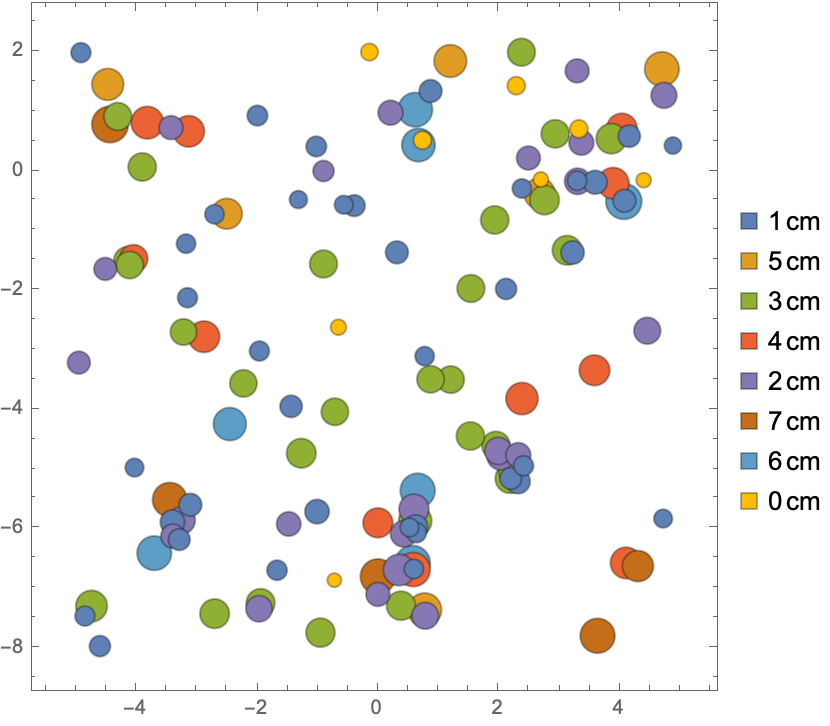### Analysis (5)

Compute probability of finding a point within given radius of an existing point - NearestNeighborG is the CDF of the nearest neighbor distribution:

 In:=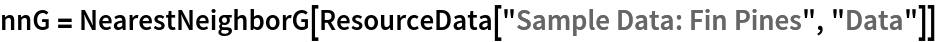Out=In:=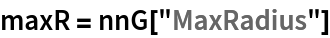Out=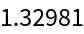In:=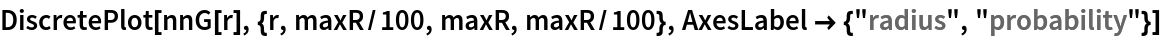Out=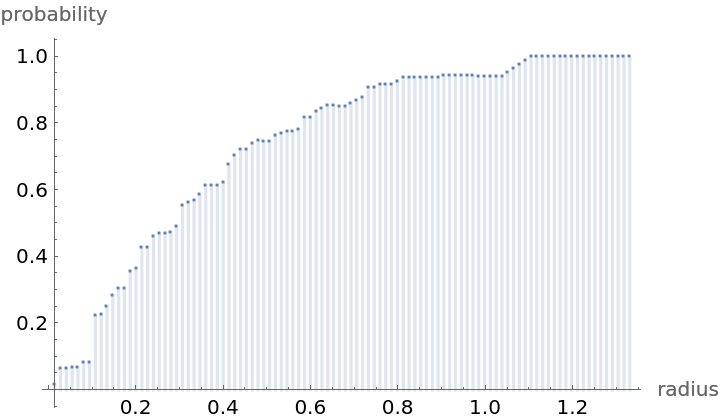NearestNeighborG as the CDF of nearest neighbor distribution can be used to compute the mean distance between a typical point and its nearest neighbor - the mean of a positive support distribution can be approximated via a Riemann sum of 1- CDF. To use Riemann approximation create the partition of the support interval from 0 to maxR into 100 parts and compute the value of the NearestNeighborG at the middle of each subinterval:

 In:=Now compute the Riemann sum to find the mean distance between a typical point and its nearest neighbor:

 In:=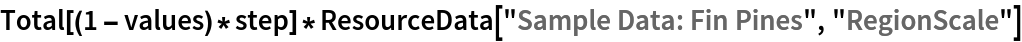Out=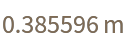Test for complete spacial randomness:

 In:=Out=Fit a Poisson point process to data:

 In:=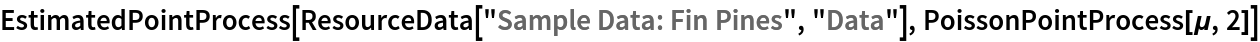Out=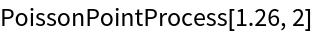Gosia Konwerska, "Sample Data: Fin Pines" from the Wolfram Data Repository (2022)